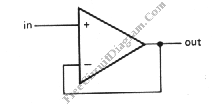## Differentiator Operator with Op-Amp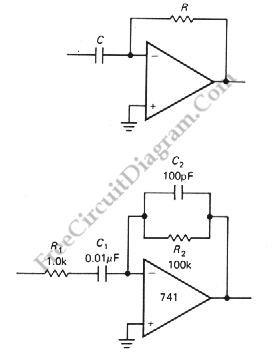Another application of OP-AMP is differentiator. Differentiator circuit produce an output if the input is changing. The output can positive or negative, depends on the change of the input: whether it’s decreasing or increasing.  This circuit is a bias stable. The current is produced by the rate of change of input voltage because the inverting input is grounded. Here is […]

## Summing Amplifier with Op-Amp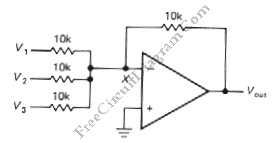One of inverting amplifier application is for summing several electric signals. The output of this circuit produce the sums of the input voltages.  We can use both positive or negative voltage for the inputs. The input resistors doesn’t have to be  same values. The input can be expanded to several digits. Since the point X is a virtual ground, the […]

## Differential Amplifier with Op-Amp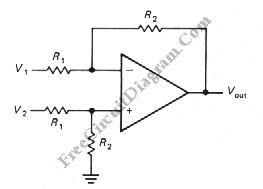The schematic diagram below shows a differential amplifier circuit. This circuit is used to get a common-mode rejection ratios. This circuit need a precise resistor matching to get common-mode rejection ratios because the current source uses matched resistor ratios. Here is the circuit: The gain of this circuit is determined by following equation: gain=R2/R1 The Vout is determined by following […]

## LM311 Comparator with Hysteresis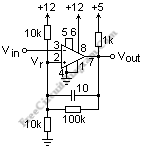An LM311 comparator circuit with the pin numbers  is shown in the schematic diagram figure below. The ground for this transistor is at pin 1 and the open collector output is at pin 7. It is shown pulled up to the logic voltage of +5 with a 1k resistor, which is typical. The LM311 output can sink 8 mA. A […]

## Noninverting Amplifier with Op-Amp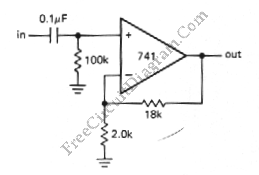This is a noninverting amplifier circuit. This circuit amplify the input voltage with the same phase with output voltage. This circuit has infinite input impedance, but using 741, the input impedance would be hundreds of megaohms. The output impedance is a little, in fraction of an ohm. Here is the schematic diagram of the circuit: This circuit is made for […]

## Inverting Amplifier with Op-Amp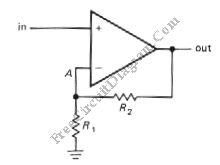This is a Inverting amplifier circuit. This circuit is used to amplify the application that requires large(closed-loop) voltage gain since it has low input impedance. The output of this circuit is  an amplified signal with different phase wit the input. This circuit uses a feedback. Here is the schematic diagram of the circuit: The R1 is 10K and R2 is […]